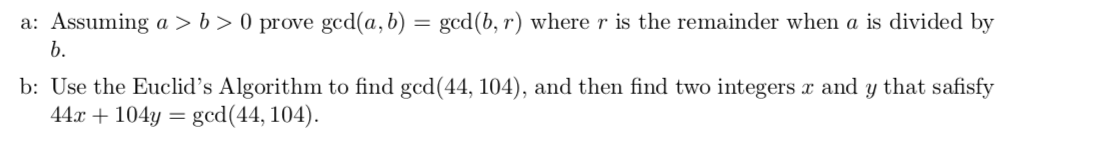# a: Assuming a > b > 0 prove gcd(a, b) = gcd(b, r) where r is the remainder when a is divided byb.b: Use the Euclid's Algorithm to find gcd(44, 104), and then find two integers æ and y that safisfy44.x104y=gcd(44, 104)

Question
2 views

solve part bhelp_outlineImage Transcriptionclosea: Assuming a > b > 0 prove gcd(a, b) = gcd(b, r) where r is the remainder when a is divided by b. b: Use the Euclid's Algorithm to find gcd(44, 104), and then find two integers æ and y that safisfy 44.x104y= gcd(44, 104) fullscreen
check_circle

Step 1

Let the number a and b can be written as a = b q + r where r lies between 0 and a.

Now we have to prove that gcd (a, b) = gcd (b, r)

GCD of two numbers a and b is defined as the biggest number which divides both a as well as b, denoted by gcd (a, b).

Now a = b q + r, then d is the common divisor between a and b,

Step 2

Thus d is the common divisor of r and b,

Now d is the common divisor of r and b. Then:

...

### Want to see the full answer?

See Solution

#### Want to see this answer and more?

Solutions are written by subject experts who are available 24/7. Questions are typically answered within 1 hour.*

See Solution
*Response times may vary by subject and question.
Tagged in

### Math